# How to use the RATE function in Excel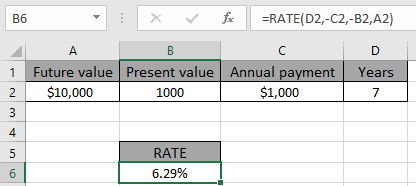In this article, we will learn How to use the RATE function in Excel.

What is the interest rate and how to calculate interest rate for a loan amount

You are already aware about the present value and future value of an amount. For example a loan of \$10,000 is to be paid on the monthly payment of \$190.  This change occurs on the periodic payment and a specific percentage. This specific percentage is called the interest rate (nominal rate). Higher the rate, higher will the future value of the amount. Lend money on more interest rate and borrow money on less interest.

RATE Function in Excel

RATE function is used to find the Interest rate of the data set in Excel. It requires the following arguments to calculate the interest rate.

Syntax:

 = RATE (nper, pmt, pv, [fv], [type], [guess])

nper: total no. of payment period.

pmt: amount paid each period.

pv - The present value of future payments must be entered as a negative number.

fv: [optional] due future value. Default is 0

type - [optional] When payments are due. Default is 0.

Example :

All of these might be confusing to understand. Let's understand how to use the function using an example. Here we have data having a present value of \$10,000 and amount is paid every month till 60 months (12 years).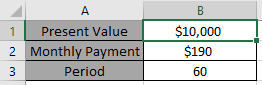We need to find the interest rate on the data provided.

Use the formula

 = RATE ( B3 , B2 , - B1 ) * 12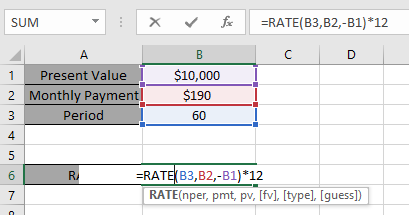Here all the references are given as cell reference as argument to the function.

Press Enter.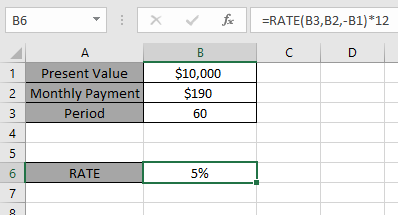The interest rate for the data set is 5%. So it means the interest rate of 5% is paid for the data provided.

Another Example

Now we will consider one more scenario to Calculate annuity for Interest rate.

Here we are given Future value \$10000, Present value \$1000, annual payment \$1000 & period of payment is till 7 years.

We need to find the interest rate on the data provided.

Use the formula

 =RATE ( D2 , - C2 , - B2 , A2 )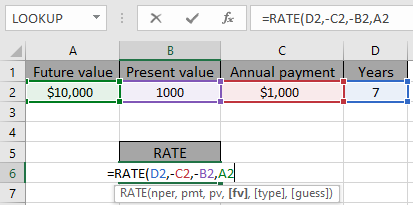Here all the references are given as cell reference as argument to the function. Negative sign is considered with annual payment & present value because credit amount & debit Amount cannot have the same sign.

Press Enter.The interest rate for the data set is 6.29 %. So it means the interest rate of 6.29 % is paid for the data provided.

Notes:

1. The function provides the #VALUE! error if any argument to the function is non - numeric.
2. The function returns #NUM! error if the credit amount & debit amount signs are not correct. Excel understands negative as amount received and positive as amount paid.
3. RATE function calculates results by iteration and can have zero or more solutions. If the successive results of RATE do not converge to within 0.0000001 after 20 iterations, The function returns the #NUM! error value.

Hope this article about How to use the RATE function in Excel is explanatory. Find more articles on financial values and related Excel formulas here. If you liked our blogs, share it with your friends on Facebook. And also you can follow us on Twitter and Facebook. We would love to hear from you, do let us know how we can improve, complement or innovate our work and make it better for you. Write to us at info@exceltip.com.

Related Articles :

How to use the MIRR function in excel : returns the Modified interest rate of return for the financial data having Investment, finance rate & reinvestment_rate using the MIRR function in Excel.

How to use the XIRR function in excel : returns the Interest rate of return for irregular interval using the XIRR function in Excel

Excel PV vs FV function : find Present Value using PV function and future value using FV function in Excel.

How to use the RECEIVED function in excel : calculates the amount which is received at maturity for a bond with an initial investment (security) and a discount rate, there are no periodic interest payments using the RECEIVED function in excel.

How to use the NPER function in excel : NPER function to calculate periods on payments in Excel.

How to use the PRICE function in excel : returns the price per \$100 face value of a security that pays periodic interest using the PRICE function in Excel.

Popular Articles :

How to use the IF Function in Excel : The IF statement in Excel checks the condition and returns a specific value if the condition is TRUE or returns another specific value if FALSE.

How to use the VLOOKUP Function in Excel : This is one of the most used and popular functions of excel that is used to lookup value from different ranges and sheets.

How to use the SUMIF Function in Excel : This is another dashboard essential function. This helps you sum up values on specific conditions.

How to use the COUNTIF Function in Excel : Count values with conditions using this amazing function. You don't need to filter your data to count specific values. Countif function is essential to prepare your dashboard.

Terms and Conditions of use

The applications/code on this site are distributed as is and without warranties or liability. In no event shall the owner of the copyrights, or the authors of the applications/code be liable for any loss of profit, any problems or any damage resulting from the use or evaluation of the applications/code.